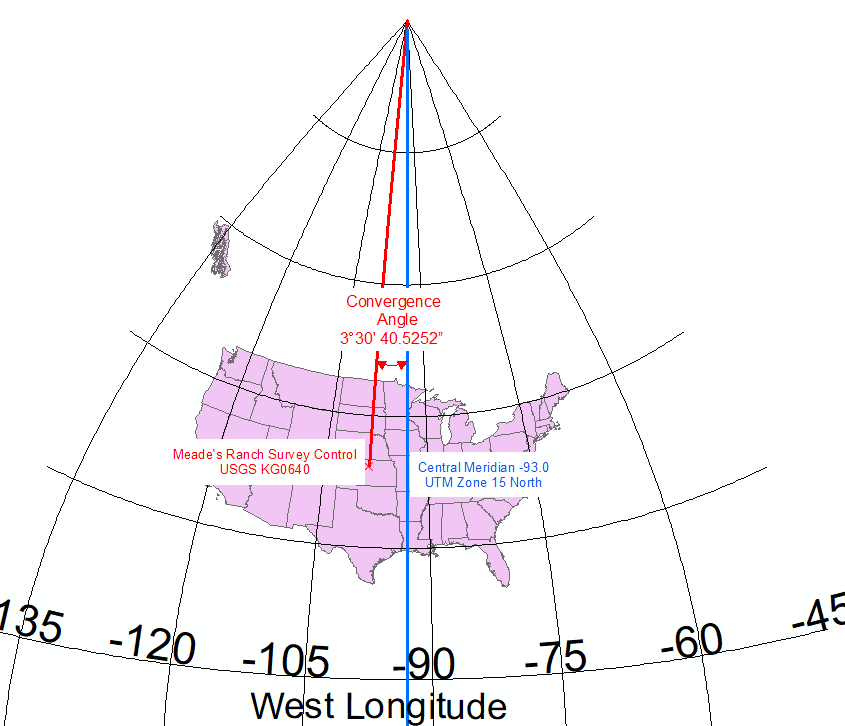English

# FAQ: What is a Grid Convergence Angle?

## Question

What is a Grid Convergence Angle?

The Calculate Convergence Angle tool calculates the angle between the Central Meridian of the projected coordinate system applied to the data, and the line which intersects the point and runs from the point to the North (or South) Pole.  A Convergence Angle between the Central Meridian of UTM Zone 15 North and the USGS Survey Control Point Meade's Ranch is shown in the following illustration.```Note: